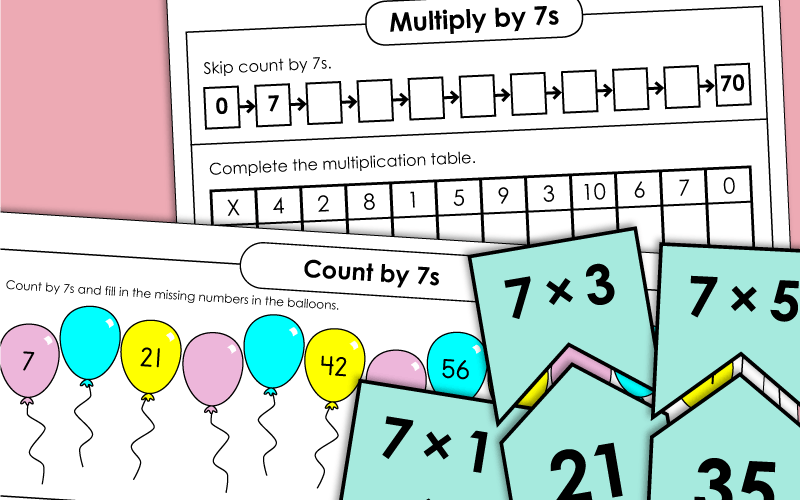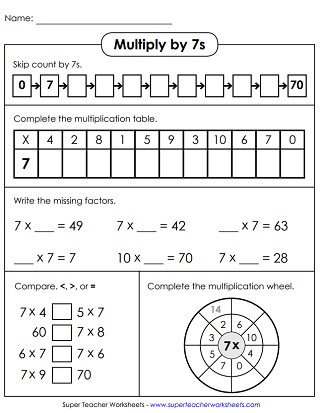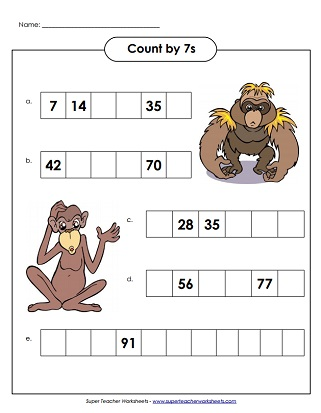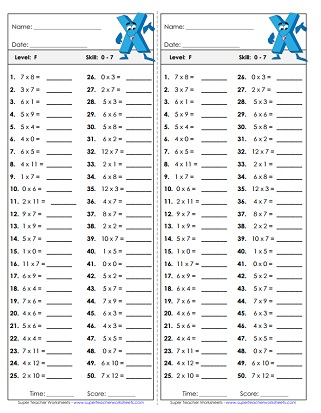# Multiplying by 7

Here you'll find basic multiplication facts up to 7, as well as worksheets with only 7s. We also have skip counting practice sheets as well.## Multiplication by7s Only

This worksheet has a skip counting number line, missing factor problems, a multiplication wheel, comparing questions, and a number table.
Multiply by 7s and write the answers. Then use the key at the bottom to determine how each section should be colored. Mystery picture is a colorful castle with flags.
Practice multiplying by 7 with these task cards, which can be used for various activities such as peer practice, scavenger hunts, class games, and more.
One way to have students use this puzzle is to "find their partner". Give each student one puzzle piece. Half of the students will have a multiplication fact and the other half will have the answers. Have them find their "partner" with the matching piece.
In this printable math activity, children will multiply by 7s in order to fill in the blank bubbles on the multiplication caterpillar.
Cut and fold to make an origami fortune teller game (aka cootie catcher). Learn and practice basic facts with factors of 7.
Practice multiplication facts with 7 as a factor. This file includes 11 flash cards, 5 super challenge cards, a sorting mat, and a self-quiz.
Print this file on heavy card stock. The cut out the airplane picture and the math fact strips. Place the fact strips through the airplane and pull to scroll up and down. Students read the facts and say the answers.

## Skip Count by 7s

Answer the critical thinking questions and solve the word problems.
0, 7, 14,... what is next? Skip count by 7s along the number line to find out.
Count by 7s to complete the dot-to-dot. The picture is a pig. Then solve the multiplication facts at the bottom of the page.
Count by 7s. Write the numbers on the penguins' bellies. Numbers go all the way up to 161.
Count by 7s and write the missing numbers on the balloons. These numbers go up to 168.
Complete the grids by filling in the missing numbers. This worksheet has a monkey theme.

## All Facts0s through 7s

On this drill worksheet, your students will review multiplication facts with factors of 0, 1, 2, 3, 4, 5, 6, and 7. Facts through 10s, up to 7x10.
This drill worksheet has all basic facts for multiplying by 0, 1, 2, 3, 4, 5, 6, and 7. Facts include 11s and 12s, up to 7x12.
This timed quiz has 50 problems on it. Covers all basic facts for 0s, 1s, 2s, 3s, 4s, 5s, 6s, and 7s. Facts through 12, up to 7x12.
Multiplication Basic Facts: 0-10

Here you'll find lots more multiplication worksheets, games, and activities for all basic facts up to 10x10.

Multiplication/Division
Fact Families

This page has lots of worksheets with multiplication and division fact families.

Multiplication by 3s

Basic facts with factors of 3 are the focus on these printable learning resources.

Multiplication by 4s

These worksheets focus specifically on the 4s times tables.

Multiplication by 5s

Here are the activities for learning multiplication facts from 5x0 up to 5x12.

Multiplication by 6s

Multiplication by 8s

Practice multiplying by the number 8. Includes mystery pictures, skip counting lessons, and more.

Multiplication by 9s

## Pictures of Our WorksheetsMy Account
Site Information